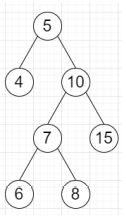# Program to find the kth smallest element in a Binary Search Tree in Python

Suppose we have a binary search tree, and another integer k we have to find the kth smallest value in the tree.

So, if the input is likek = 3, then the output will be 7

To solve this, we will follow these steps −

• stack := an empty stack

• i := 0

• ans := -1

• while stack is not empty or root is not null, do

• while root is not null, do

• push root into stack

• root := left of root

• v := pop element from stack

• if i is same as k, then

• ans := value of v

• come out from loop

• root := right of v

• i := i + 1

• return ans

Let us see the following implementation to get better understanding −

## Example

Live Demo

class TreeNode:
def __init__(self, value):
self.val = value
self.left = None
self.right = None

class Solution:
def solve(self, root, k):
stack = []
i = 0
ans = -1
while stack or root:
while root:
stack.append(root)
root = root.left
v = stack.pop()
if i == k:
ans = v.val
break
root = v.right
i += 1
return ans
ob = Solution()
root = TreeNode(5)
root.left = TreeNode(4)
root.right = TreeNode(10)
root.right.left = TreeNode(7)
root.right.right = TreeNode(15)
root.right.left.left = TreeNode(6)
root.right.left.right = TreeNode(8)
print(ob.solve(root, 3))

## Input

root = TreeNode(5)
root.left = TreeNode(4)
root.right = TreeNode(10)
root.right.left = TreeNode(7)
root.right.right = TreeNode(15)
root.right.left.left = TreeNode(6)
root.right.left.right = TreeNode(8)
3

## Output

7